JEE  >  Sample BITSAT Maths Test

# Sample BITSAT Maths Test

Test Description

## 45 Questions MCQ Test BITSAT Mock Tests Series & Past Year Papers | Sample BITSAT Maths Test

Sample BITSAT Maths Test for JEE 2023 is part of BITSAT Mock Tests Series & Past Year Papers preparation. The Sample BITSAT Maths Test questions and answers have been prepared according to the JEE exam syllabus.The Sample BITSAT Maths Test MCQs are made for JEE 2023 Exam. Find important definitions, questions, notes, meanings, examples, exercises, MCQs and online tests for Sample BITSAT Maths Test below.
Solutions of Sample BITSAT Maths Test questions in English are available as part of our BITSAT Mock Tests Series & Past Year Papers for JEE & Sample BITSAT Maths Test solutions in Hindi for BITSAT Mock Tests Series & Past Year Papers course. Download more important topics, notes, lectures and mock test series for JEE Exam by signing up for free. Attempt Sample BITSAT Maths Test | 45 questions in 55 minutes | Mock test for JEE preparation | Free important questions MCQ to study BITSAT Mock Tests Series & Past Year Papers for JEE Exam | Download free PDF with solutions
 1 Crore+ students have signed up on EduRev. Have you?
Sample BITSAT Maths Test - Question 1

### What is the area under the curve y = |x| + | x - 1| between x = 0 and x = 1 ?

Sample BITSAT Maths Test - Question 2

### The greatest term in the expansion of (3 + 2x)9, when x = 1, is

Detailed Solution for Sample BITSAT Maths Test - Question 2 The greatest term of any expansion is its middle termIn (a+x) ^n the middle term will be (n+2)/2 if n is even and if n is odd then there will be two middle terms that is (n+1)/2 and (n+3)/2 Here n is odd therefore the greatest term will be 4 and 5
Sample BITSAT Maths Test - Question 3

### The coordinates of the pole of the line lx+my+n=0 with respect to the circle x2+y2=1 are

Detailed Solution for Sample BITSAT Maths Test - Question 3

Solution :- Let (x1,y1) be the pole of the line lx+my+n=0 with respect to the hyperbola x2a2−y2b2=1. Then, the equation of the polar is

xx1/ + yy1/ = 1………(i)

Since, (x1,y1) is the pole of the line lx+my+n=0. So, the polar of (x1,y1) is also the line

lx+my+n=0...(ii)

clearly, (i) and (ii) represent the same line. Therefore,

x1(l) = y1(m) = 1/(−n)

⇒x1 = −l/n,  y1 = -m/n.

Hence, the pole of the given line with respect to the given hyperbola is

(−l/n, -m/n)

Sample BITSAT Maths Test - Question 4

If the line 2x - y + k = 0 is a diameter of the circle x2 + y2 + 6x -6y + 5 =0, then k is equal to

Detailed Solution for Sample BITSAT Maths Test - Question 4 If the given line 2x-y+k=0 is the diameter of the circle then it passes through the centre of the given circle. Which on comparing we get as (-3,3).On substituting this in the line equation we get 2(-3)- 3+k=0=> -9+k=0 K=9.
Sample BITSAT Maths Test - Question 5

If z = i log(2 - √3), then cos z =

Sample BITSAT Maths Test - Question 6

The differential equation of the family of lines passing through the origin is

Detailed Solution for Sample BITSAT Maths Test - Question 6

The equation of line passing through the origin is y = mx , when m is constant
Diffrence w.r.t x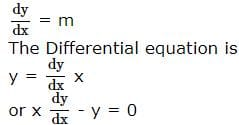Sample BITSAT Maths Test - Question 7

Which of the following is a solution of the differential equation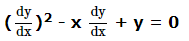Detailed Solution for Sample BITSAT Maths Test - Question 7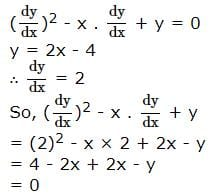Sample BITSAT Maths Test - Question 8

If y' = x-y/x+y, then its solution is

Detailed Solution for Sample BITSAT Maths Test - Question 8

dy/dx= x−y/x+y

Put,y=vx

⟹dy/dx=v+x(dv/dx)

⟹v+xdv/dx=1−v/1+v

⟹xdv/dx = 1−2v−v^2/(1+v)

⟹∫v+1/(v+1)^2−2dv=−∫1/xdx

⟹1/2ln⁡|[(v+1)^2−2]| = 2ln⁡|c1/x|

⟹x^2(v^2+2v−1)=C

Where C = 2ln⁡|c1/x|

Since,v=y/x, we get

⟹ y2+2xy−x2=C

Sample BITSAT Maths Test - Question 9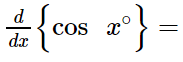Detailed Solution for Sample BITSAT Maths Test - Question 9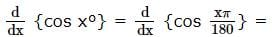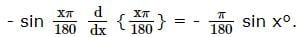Sample BITSAT Maths Test - Question 10

(d/dx)[tan⁻1((sinx+cosx)/(cosx-sinx))]

Detailed Solution for Sample BITSAT Maths Test - Question 10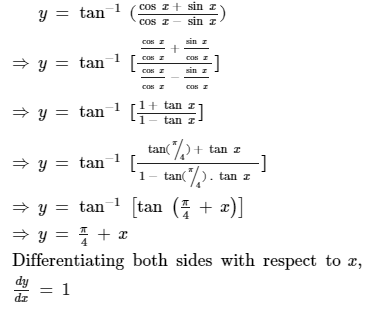Sample BITSAT Maths Test - Question 11

Value of 1 + log x + (log x)2/2! + (log x)3/3! + ..... ∞ is

Sample BITSAT Maths Test - Question 12

The angle of elevation of a cloud from a point h mt above the surface of a lake is θ and the angle of depression of its reflection in the lake is φ . The height of the cloud is

Sample BITSAT Maths Test - Question 13

The eccentricity of the conjugate hyperbola of the hyperbola x2 - 3y2 = 1 is

Sample BITSAT Maths Test - Question 14

Which of the following functions is a solution of the differential equation (dy/dx)2 - x (dy/dx) + y = 0?

Sample BITSAT Maths Test - Question 15

The solution of the differential equation (dy/dx) = (y/x) + (φ (y/x)/φ' (y/x)) is

Sample BITSAT Maths Test - Question 16

tan⁻1(1/4)+ tan⁻1(2/9) is equal to

Sample BITSAT Maths Test - Question 17

f(x) = ||x| - 1| is not differentiable at

Sample BITSAT Maths Test - Question 18

For every n ∈ N, 23n-7n-1 is divisible by

Detailed Solution for Sample BITSAT Maths Test - Question 18

Solution :- 23n−7n−1=8n−7n−1

=(7+1)n−7n−1

=C(n,0)7n+C(n,1)7n−1+...+C(n,n−2)72+C(n,n−1)71+C(n,n)70−7n−1

Now, C(n,0)7n+C(n,1)7n−1+...+C(n,n−2)72 is clearly divisible by 49.

So, we can write it as 49k.

So, our expression becomes,

=49k+C(n,n−1)71+C(n,n)70−7n−1

=49k+7n+1−7n−1

=49k

∴23n−7n−1=49k

So, clearly 23n−7n−1 is divisible by 49.

Sample BITSAT Maths Test - Question 19

If A, B are two square matrices such that AB = A and BA = B, then

Sample BITSAT Maths Test - Question 20

For a square matrix A, it is given that AA' = I, then A is a

Sample BITSAT Maths Test - Question 21

The maximum value of xy subject to x+y=8 is

Detailed Solution for Sample BITSAT Maths Test - Question 21 Because x and y should have value 4 so that 4+4=8 and 4*4=16
Sample BITSAT Maths Test - Question 22

The real value of α for which the expression 1-i sin α/1+2 i sin α is purely real is

Detailed Solution for Sample BITSAT Maths Test - Question 22
The real value of α for which the expression (1-i sin α) / (1+2 i sin α) is purelySample BITSAT Maths Test - Question 23

The angle between lines xy=0 is

Detailed Solution for Sample BITSAT Maths Test - Question 23

For xy = 0
The lines are: x = 0 & y = 0 which are the Y and X axis respectively which are perpendicular.

Sample BITSAT Maths Test - Question 24

The focus of the parabola (y-2)2=20(x+3) is

Sample BITSAT Maths Test - Question 25

The equation of the normal to the curve x2 = 4y at (1, 2) is

Sample BITSAT Maths Test - Question 26

Two finite sets have m and n elements, the total number of subsets of the first set is 56 more than the total number of subsets of the second. The value of m and n are respectively

Detailed Solution for Sample BITSAT Maths Test - Question 26

Let A denote the first set and B denote the second set
We have, n(A) = 2m and n(B) = 2n
As per the question, we have
n(A) = 56 + n(B)
⇒ n(A) - n(B) = 56
⇒ 2m - 2n = 56
⇒ 2n (2m - n - 1)
⇒ 2n (2m - n - 1) = 8 x 7
⇒ 2n = 8 = 23 or (2m - n - 1) = 7
⇒ n = 3 or 2m - n = 8 = 23 = 26 - 3
⇒ n = 3 or m - n = 3
⇒ n = 3 or m = 6
Hence, the required values of m and n are 6 and 3 respectively

Sample BITSAT Maths Test - Question 27

In how many ways can the letters of the word ARRANGE be arranged so that R's are never together?

Detailed Solution for Sample BITSAT Maths Test - Question 27

Reqd. ways =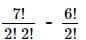= 1260 - 360 = 900

Sample BITSAT Maths Test - Question 28

A and B are events such that P(A ∪ B) = 3/4, P(A ∩ B) = 1/4, P(A̅)= 2/3, then P(A̅ ∩ B) is

Sample BITSAT Maths Test - Question 29

The probability that a number selected at random from the set of numbers {1,2,3,....,100} is a cube is

Sample BITSAT Maths Test - Question 30

In a equilateral triangle r : R : r1 is

Detailed Solution for Sample BITSAT Maths Test - Question 30

A = B = C = 60o
r : R : r1 = 4R sin (A/2) sin (B/2) sin (C/2) : R : 4R sin (A/2) cos (B/2) cos (C/2)
= 4 (1/2) (1/2) (1/2) : 1 : 4 (1/2) (√3 /2) (√3 /2) = (1/2) : 1 : (3/2) = 1 : 2 : 3

Sample BITSAT Maths Test - Question 31

The perimeter of a triangle is 16cm. One of the sides is of length 6cm. If the area of the triangle is 12sq.cm, then the triangle is

Sample BITSAT Maths Test - Question 32

The product of cube roots of -1 is equal to

Sample BITSAT Maths Test - Question 33

The sum of all 2-digit odd numbers is

Detailed Solution for Sample BITSAT Maths Test - Question 33 The two digit odd numbers
11,13,15,...., 99

a = 11, n = 45, d = 2

Sn = n/2[2a + (n-1)d}]

= 45/2 [22 + 44 × 2]

= 45/2 × 110

= 45 × 55

= 2475
Sample BITSAT Maths Test - Question 34

If the sum of first n terms of an A.P. be 3n2 - n and its common difference is 6, then its first term is

Sample BITSAT Maths Test - Question 35

In a town of 10,000 families it was found that 40% family buy newspaper A, 20% buy newspaper B, and 10% families buy newspaper C, 5% families buy A and B, 3% buy B and C and 4% buy A and C. If 2% families buy all the three newspapers, then number of families which buy A only is

Sample BITSAT Maths Test - Question 36

If f : N x N → N is such that f (m,n) = m + n where N is the set of natural numbers, then which of the following is true?

Sample BITSAT Maths Test - Question 37

The ortho centre of triangle whose vertices are (0,0), (3,0) and (0,4) is

Sample BITSAT Maths Test - Question 38

The angle between the curves y2=x at x2=y at (1,1) is

Sample BITSAT Maths Test - Question 39

The angle between the planes 2x-y+z=6 and x+y+2z=7 is

Sample BITSAT Maths Test - Question 40

The acute angle between the planes 2x-y+z=6 and x+y+2z=3 is

Sample BITSAT Maths Test - Question 41

If 2cos2x+3sinx-3=0, 0≤x≤180o, then x=

Sample BITSAT Maths Test - Question 42

The equation sinx+cosx=2 has

Sample BITSAT Maths Test - Question 43

Maximum value of cos2x+cos2y-cos2z is

Detailed Solution for Sample BITSAT Maths Test - Question 43

Max. value will occur when cosx=cosy=1 and cosz=0

Sample BITSAT Maths Test - Question 44

If 3i+4j and -5i+7j represent the sides of a triangle, then its area is

Sample BITSAT Maths Test - Question 45

If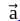and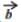are mutually perpendicular, then (a+b)2=

## BITSAT Mock Tests Series & Past Year Papers

2 videos|15 docs|70 tests
 Use Code STAYHOME200 and get INR 200 additional OFF Use Coupon Code
Information about Sample BITSAT Maths Test Page
In this test you can find the Exam questions for Sample BITSAT Maths Test solved & explained in the simplest way possible. Besides giving Questions and answers for Sample BITSAT Maths Test, EduRev gives you an ample number of Online tests for practice

## BITSAT Mock Tests Series & Past Year Papers

2 videos|15 docs|70 tests